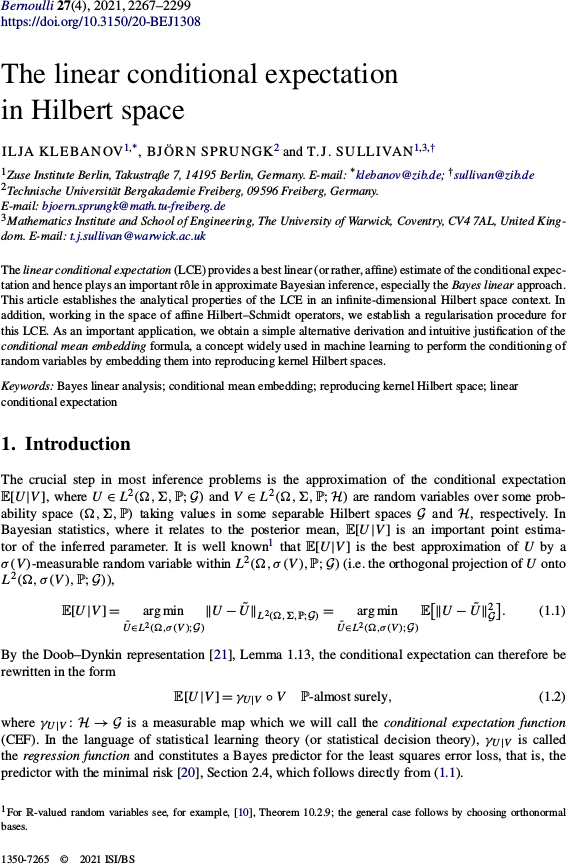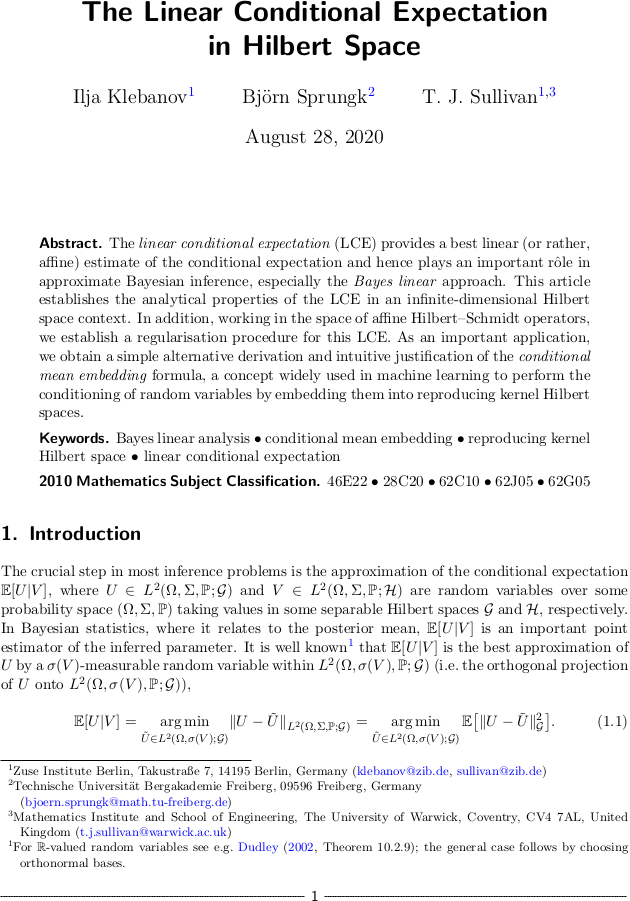# Tim Sullivan

### #sprungk

Clear Search### Dimension-independent MCMC on spheres

Han Cheng Lie, Daniel Rudolf, Björn Sprungk and I have just uploaded a preprint of our latest article, “Dimension-independent Markov chain Monte Carlo on the sphere”, to the arXiv. In this paper, motivated by problems such as Bayesian binary classification over continuous spaces, for which the parameter space is naturally an infinite-dimensional sphere of functions, we consider MCMC methods for inference on spheres of Hilbert spaces. In particular, we construct MCMC methods that have dimension-independent performance in terms of their acceptance probability, spectral gap, etc.; we also show how more naive approaches may lack basic properties such as Markovianity and reversibility; how how even sophisticated geometric MCMC approaches can still suffer from the curse of dimension.

Abstract. We consider Bayesian analysis on high-dimensional spheres with angular central Gaussian priors. These priors model antipodally-symmetric directional data, are easily defined in Hilbert spaces and occur, for instance, in Bayesian binary classification and level set inversion. In this paper we derive efficient Markov chain Monte Carlo methods for approximate sampling of posteriors with respect to these priors. Our approaches rely on lifting the sampling problem to the ambient Hilbert space and exploit existing dimension-independent samplers in linear spaces. By a push-forward Markov kernel construction we then obtain Markov chains on the sphere, which inherit reversibility and spectral gap properties from samplers in linear spaces. Moreover, our proposed algorithms show dimension-independent efficiency in numerical experiments.

Published on Wednesday 22 December 2021 at 12:00 UTC #preprint #mcmc #lie #rudolf #sprungk### Linear conditional expectation in Hilbert space in Bernoulli

The article “The linear conditional expectation in Hilbert space” by Ilja Klebanov, Björn Sprungk, and myself has just appeared in its final form in the journal Bernoulli. In this paper, we study the best approximation $$\mathbb{E}^{\mathrm{A}}[U|V]$$ of the conditional expectation $$\mathbb{E}[U|V]$$ of an $$\mathcal{G}$$-valued random variable $$U$$ conditional upon a $$\mathcal{H}$$-valued random variable $$V$$, where “best” means $$L^{2}$$-optimality within the class $$\mathrm{A}(\mathcal{H}; \mathcal{G})$$ of affine functions of the conditioning variable $$V$$. This approximation is a powerful one and lies at the heart of the Bayes linear approach to statistical inference, but its analytical properties, especially for $$U$$ and $$V$$ taking values in infinite-dimensional spaces $$\mathcal{G}$$ and $$\mathcal{H}$$, are only partially understood — which this article aims to rectify.

I. Klebanov, B. Sprungk, and T. J. Sullivan. “The linear conditional expectation in Hilbert space.” Bernoulli 27(4):2267–2299, 2021. doi:10.3150/20-BEJ1308

Abstract. The linear conditional expectation (LCE) provides a best linear (or rather, affine) estimate of the conditional expectation and hence plays an important rôle in approximate Bayesian inference, especially the Bayes linear approach. This article establishes the analytical properties of the LCE in an infinite-dimensional Hilbert space context. In addition, working in the space of affine Hilbert–Schmidt operators, we establish a regularisation procedure for this LCE. As an important application, we obtain a simple alternative derivation and intuitive justification of the conditional mean embedding formula, a concept widely used in machine learning to perform the conditioning of random variables by embedding them into reproducing kernel Hilbert spaces.

Published on Wednesday 25 August 2021 at 08:00 UTC #publication #tru2 #bayesian #rkhs #mean-embedding #klebanov #sprungk### Linear conditional expectation in Hilbert space

Ilja Klebanov, Björn Sprungk, and I have just uploaded a preprint of our recent work “The linear conditional expectation in Hilbert space” to the arXiv. In this paper, we study the best approximation $$\mathbb{E}^{\mathrm{A}}[U|V]$$ of the conditional expectation $$\mathbb{E}[U|V]$$ of an $$\mathcal{G}$$-valued random variable $$U$$ conditional upon a $$\mathcal{H}$$-valued random variable $$V$$, where “best” means $$L^{2}$$-optimality within the class $$\mathrm{A}(\mathcal{H}; \mathcal{G})$$ of affine functions of the conditioning variable $$V$$. This approximation is a powerful one and lies at the heart of the Bayes linear approach to statistical inference, but its analytical properties, especially for $$U$$ and $$V$$ taking values in infinite-dimensional spaces $$\mathcal{G}$$ and $$\mathcal{H}$$, are only partially understood — which this article aims to rectify.

Abstract. The linear conditional expectation (LCE) provides a best linear (or rather, affine) estimate of the conditional expectation and hence plays an important rôle in approximate Bayesian inference, especially the Bayes linear approach. This article establishes the analytical properties of the LCE in an infinite-dimensional Hilbert space context. In addition, working in the space of affine Hilbert–Schmidt operators, we establish a regularisation procedure for this LCE. As an important application, we obtain a simple alternative derivation and intuitive justification of the conditional mean embedding formula, a concept widely used in machine learning to perform the conditioning of random variables by embedding them into reproducing kernel Hilbert spaces.

Published on Friday 28 August 2020 at 09:00 UTC #preprint #tru2 #bayesian #rkhs #mean-embedding #klebanov #sprungk